# Texas Go Math Grade 2 Lesson 13.1 Answer Key Even and Odd Numbers

Refer to our Texas Go Math Grade 2 Answer Key Pdf to score good marks in the exams. Test yourself by practicing the problems from Texas Go Math Grade 2 Lesson 13.1 Answer Key Even and Odd Numbers.

## Texas Go Math Grade 2 Lesson 13.1 Answer Key Even and Odd Numbers

Explore

Use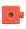to show each number.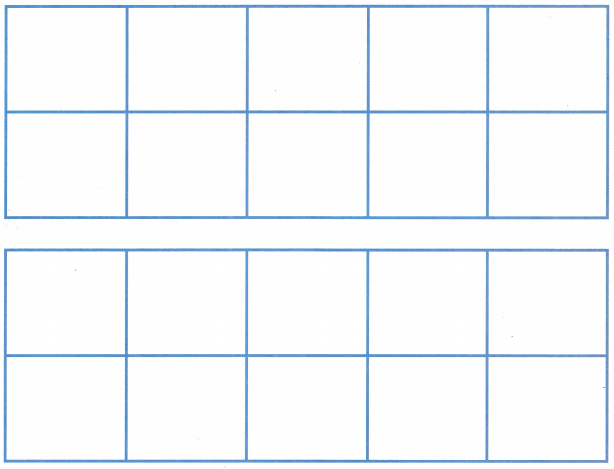FOR THE TEACHER • Read the following problem. Beca has 8 toy cars. Can she arrange her cars in pairs on a shelf? Have children set pairs of cubes vertically on the ten frames. Continue the activity for the numbers 7 and 10.

Math Talk
Mathematical Processes

When you make pairs for 7 and for 10. how are these models different? Explain.
If we make a pair for 7 then we can see that there will be 3 cubes left and when we make a pair of 10 then we can see no cube left.

Model and Draw

Count out cubes for each number. Make pairs. Even numbers show pairs with no cubes left over. Odd numbers show pairs with one cube left over.5 is an odd number,
8 is an even number,
12 is an even number,
15 is an odd number.

Explanation:
Here,
5 is an odd number, as there is one cube left in the pair.
8 is an even number, as there is no cube left in the pair.
12 is an even number, as there is no cube left in the pair.
15 is an odd number. as there is one cube left in the pair.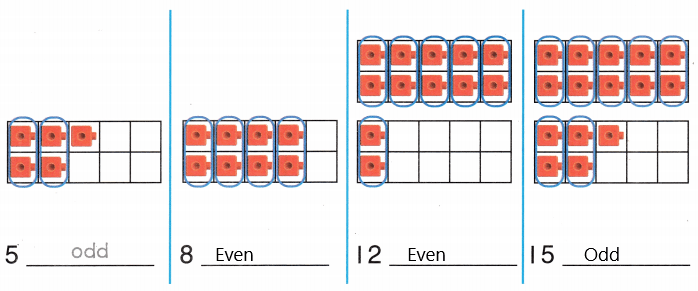Share and Show

Use cubes. Count out the number of cubes. Make pairs. Then write even or odd.

Question 1.
10 _____________
10 is an even number.

Explanation:
Here, the number 10 is an even number, as there will be no cube left in the pair.Question 2.
13 _____________
13 is an odd number.

Explanation:
Here, the number 13 is an odd number, as there will be one cube left in the pair.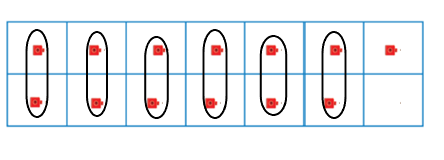Question 3.
18 _____________
18 is an even number.

Explanation:
Here, the number 18 is an even number, as there will be no cube left in the pair.

Question 4.
19 _____________
19 is an odd number.

Explanation:
Here, the number 19 is an odd number, as there will be one cube left in the pair.

Question 5.
14 _____________
14 is an even number.

Explanation:
Here, the number 14 is an even number, as there will be no cube left in the pair.

Question 6.
22 _____________
22 is an even number.

Explanation:
Here, the number 22 is an even number, as there will be no cube left in the pair.

Question 7.
23 _____________
23 is an odd number.

Explanation:
Here, the number 23 is an odd number, as there will be one cube left in the pair.

Question 8.
29 _____________
29 is an odd number.

Explanation:
Here, the number 29 is an odd number, as there will be one cube left in the pair.

Question 9.
21 _____________
21 is an odd number.

Explanation:
Here, the number 21 is an odd number, as there will be one cube left in the pair.

Question 10.
26 _____________

26 is an even number.

Explanation:
Here, the number 26 is an even number, as there will be no cube left in the pair.

Problem Solving

Shade in the ten frames to show the number. Circle even or odd.

Question 11.25 is an odd number.

Explanation:
Given that the number is 25, so the given number is an odd number as there will be one cube left in the pair.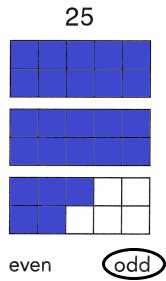Question 12.30 is an even number.

Explanation:
Given that the number is 30, so the given number is an even number as there will be no cube left in the pair.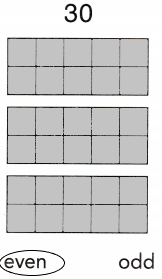Question 13.28 is an even number.

Explanation:
Given that the number is 28, so the given number is an even number as there will be no cube left in the pair.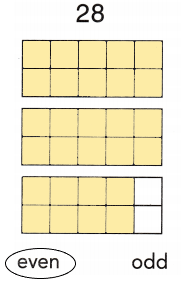Solve

Question 14.
H.O.T. Multi-Step Choose an even number between 25 and 34. Draw a picture and then explain why it is an even number.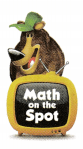30 is an even number.

Explanation:
Here, we need to choose an even number between 25 and 34. As we need to choose an even number, so we will choose 30. 30 is an even number as there will be no cube left in the pair.Question 15.
H.O.T. Make a list of 2-digit numbers that have a 3 in the ones place. Circle the numbers that are odd numbers.
23,33,43,53,63.

Explanation:
The list of 2-digit numbers that have a 3 in the ones place is
23,33,43,53,63.
All the numbers are odd numbers as they are not divisible by 2.

Question 16.
Connect Dixon has an even number of socks. Which of these could be the number of socks that he has?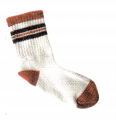111019
10.

Explanation:
Here, in the given options 10 is an even number. As 10 is divisible by 2.

Question 17.
Multi-Step Bill has an even number of gloves Pam has an odd number of mittens. Which could be their gloves and mittens?12 gloves and 9 mittens14 gloves and 12 mittens13 gloves and 8 mittens
12 gloves and 9 mittens.

Explanation:
Given that Bill has an even number of gloves Pam has an odd number of mittens, so it could be 12 gloves and 9 mittens. As 12 is an even number and 9 is an odd number.

Question 18.
Use cubes. Count out 17 cubes. Make pairs. Then write even or odd. Draw a picture to show what you did.
17 _____________
17 is an odd number.

Explanation:
Here, number 17 is an odd number, as we can see in the picture there is one cube left.Question 19.
Texas Test Prep There are an even number of girls and an odd number of boys in Gina’s class. Which of these choices could tell about her class?9 girls and 8 boys10 girls and 7 boys11 girls and 9 boys
10 girls and 7 boys.

Explanation:
Given that, there are an even number of girls and an odd number of boys in Gina’s class. So it could be 10 girls and 7 boys, as 10 is an even number and 7 is an odd number.

### Texas Go Math Grade 2 Lesson 13.1 Homework and Practice Answer Key

Shade in the ten frames to show the number. Circle even or odd.

Question 1.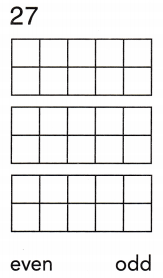27 is an odd number.

Explanation:
Here, number 27 is an odd number, as we can see in the picture there is one cube left.Question 2.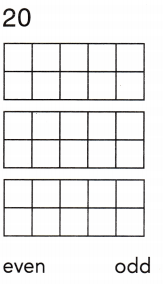20 is an even number.

Explanation:
Given that the number is 20, so the given number is an even number as there will be no cube left in the pair.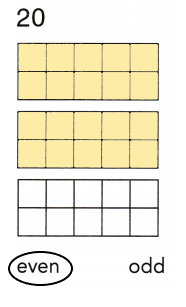Question 3.24 is an even number.

Explanation:
Given that the number is 24, so the given number is an even number as there will be no cube left in the pair.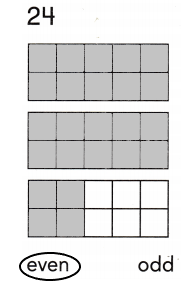Problem Solving

Question 4.
Make a list of the 2-digit numbers that have a 6 in the ones place. Circle the numbers that are even.
26,36,46,56,66.

Explanation:
The list of 2-digit numbers that have a 6 in the ones place is
26,36,46,56,66.
All the numbers are even numbers as they are divisible by 2.

Question 5.
Multi-Step Choose an even number between 24 and 35. Draw a picture and explain why it is an even number.
28 is an even number.

Explanation:
Here, we need to choose an even number between 24 and 35. As we need to choose an even number, so we will choose 28. 28 is an even number as there will be no cube left in the pair.Lesson Check

Question 6.
There are an even number of boys and an odd number of girls in Lena’s class. Which could tell about her class?There are 9 boys and 12 girls.There are 10 boys and 9 girls.There are 11 boys and 13 girls.
There are 10 boys and 9 girls.

Explanation:
Given that there are an even number of boys and an odd number of girls in Lena’s class. So it could be 10 boys and 9 girls as 10 is an even number and 9 is an odd number.

Question 7.
Amber has an odd number of shoes. Which of these could be the number of shoes that she has?181512
15.

Explanation:
Given that Amber has an odd number of shoes. So the number of shoes that she has is 15 as 15 is an odd number.

Question 8.
Terry has an even number of dinner plates. Which of these could be the number of plates that he has?8711
8.

Explanation:
Given that Terry has an even number of dinner plates. So the number of plates that he has is 8, as 8 is an even number.

Question 9.
Together, Jan and her brother have an odd number of pencils and an even number of crayons. Which could be their pencils and crayons?12 pencils and q crayons14 pencils and 12 crayons13 pencils and 8 crayons